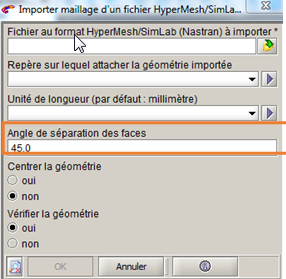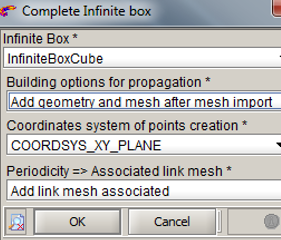# Flux mesh import workflow

## Introduction

This section presents the steps to follow in Flux to import the mesh through the Nastran file.

## Workflow

In the following table, the steps to follow in Flux for a nominal scenario are presented.

Stage Description Illustration
1 Open Flux, out of the Modeler context
2

Import the mesh

Project > Import > Import mesh > Import mesh from a HyperMesh/SimLab/OptiStruct (Nastran) file

• Select the Nastran (or OptiStruct) file to import
• Define the coordinate system and units if necessary
• Change the « face split angle » if necessary: this angle corresponds to the minimal angle of facets* separation (two facets defining an angle superior or equal to this value will be separated to two faces. For an inferior angle, the facets are merged).
• Center or not the geometry
• Verify or not the geometry

That allows doing a « fast & quick » check of the geometry defaults: superimposed points, intersections between lines. To make a more complete check, launch the command:

Geometry/Check geometry

→ After the import, we recover in Flux :

• The mesh elements
• A « mesh object » containing the imported mesh
• The lines, faces from the facets merge and the volumes from SimLab/HyperMesh components
• The volume regions from the components
Note: * a facet corresponds to an elementary face defined by a mesh surface element3

It is possible to create the infinite box in Flux after mesh import (if air box has not been create in SimLab or HyperMesh):

• Create infinite box: Physics > Infinite box > New
• Complete infinite box to generate the air volume and the associated mesh:

Physics > Infinite box > Complete infinite box

Choose the option : « Add geometry and mesh after mesh import »